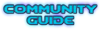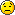Trying to Understand FloorPlane

Moderator: GZDoom Developers

Forum rules
Before asking on how to use a ZDoom feature, read the ZDoom wiki first. If you still don't understand how to use a feature, then ask here.

Trying to Understand FloorPlane

Hi, new mapper here, trying to understand slopes. I've got the AlignFloor working, but there are some situations where I can't use it. So I'm looking at sector FloorPlane equations. But what I'm seeing doesn't make sense.

So I start by making a square room, 128x128, around the origin, so from (-64,64) to (64,-64). I want to make a slope that is at flat 0 on the south wall and rises to 128 on the north wall.
So three points I could use are the two on the south floor, (-64,-64,0) and (64,-64,0). Then I can pick a point on north wall, so (-64,64,128)
I plug these three coordinates into a slope plane calculator and I get this out:
0x + -16384y + 15104z + -1048576 = 0
So I put this into my sector like this:
floorplane_a = 0;
floorplane_b = -16384;
floorplane_c = 15104;
floorplane_d = -1048576;
I notice these numbers look kinda big, and they make a ramp that is way high off the map. But I plug all three coordinates into that equation and it checks out, so what's the deal?

So I manually create the same ramp in UBD, using 0,45,64 as my rotation, angle, and offset. That gives me the ramp I want. I take a peek at the TEXTMAP and see this:
floorplane_a = 0.0;
floorplane_b = -0.707106781186547;
floorplane_c = 0.707106781186548;
floorplane_d = -45.2548339959391;

Very different numbers from what I got, and plugging those constants into the equation with any of those points doesn't check out. So is there a different equation in use or what?

Unfortunately I don't understand a lot about 3d math, I just want to know what equation I can plug in to get those a,b,c,d values outSir Robin

Joined: 22 Dec 2021
Operating System: Windows 10/8.1/8/201x 64-bit
OS Test Version: No (Using Stable Public Version)
Graphics Processor: Intel (Modern GZDoom)

Re: Trying to Understand FloorPlane

Thanks to boris, the heavy lifting is already done for you when you use Ultimate Doom Builder.

Depending on how you want to slope the sectors you could use the Visual Editing Mode to either

• slope sectors for simple to complex slopes
• slope vertices for more complex slopes
(triangulate the sectors first: see Draw Grid Mode - Draw Setting side bar)
• slope 3D floors

See F5 to set keys for the actions
Spoiler:

videos by bridgeburnerKappes BuurJoined: 17 Jul 2003

Re: Trying to Understand FloorPlane

Hi, thanks for the suggestion. Yes I can create the ramps in UDB and get them the way I want them. But I've written a script to generate levels for me. I give it parameters on how I want the level to look and it generates lists of vertices and lines and sectors and dumps those all into a TEXTMAP file. It takes care of all the nitty gritty stuff for me like lining up all the textures so I don't have to do all that by hand. Right now everything works except the slopes. So that's why I'm trying to understand what formulas I can use to generate those programmatically.

The link you posted to the DRD site, I notice there is a link for Forum which leads to a Development Build forums. Would that be a good place to ask this question?Sir Robin

Joined: 22 Dec 2021
Operating System: Windows 10/8.1/8/201x 64-bit
OS Test Version: No (Using Stable Public Version)
Graphics Processor: Intel (Modern GZDoom)

Re: Trying to Understand FloorPlane

Aha, I see. You want to do this the math way.
There is a difference between using a math calculator to get the vectors that way and the way GZDoom does the coding fu to make the result look correct.

The best persons to ask would be the GZDoom dev team, Graf Zahl, Rachael and boris, the developer/maintainer of UDB.

I would think that the best forums to ask your questions would be here in Mapping or over at Creation, Conversion, and Editing - Ultimate Doom Builder.

Take a look at the source code
https://github.com/coelckers/gzdoom
https://github.com/jewalky/UltimateDoomBuilder
I took a gander at them, but this goes right over my head.Kappes BuurJoined: 17 Jul 2003

Re: Trying to Understand FloorPlane

Sir Robin wrote:x + -16384y + 15104z + -1048576 = 0

Are you sure you used the correct formula/values? With the points you gave it should be

0x + -16384y + 16384z + -1048576 = 0

The difference between that and what UDB stores is simply that the values in UDB are normalized.

Here's how UDB computes a plane equation from 3 points: https://github.com/jewalky/UltimateDoom ... cs#L81-L89
boris
I post less than Manc and Hobo

Joined: 15 Jul 2003

Re: Trying to Understand FloorPlane

boris wrote:
Sir Robin wrote:x + -16384y + 15104z + -1048576 = 0

Are you sure you used the correct formula/values? With the points you gave it should be

0x + -16384y + 16384z + -1048576 = 0

The difference between that and what UDB stores is simply that the values in UDB are normalized.

Here's how UDB computes a plane equation from 3 points: https://github.com/jewalky/UltimateDoom ... cs#L81-L89

Whoops, good spot. It was correct in my code, I must have fudged it somewhere in copy-pasting here. Even with the proper values in, the ramp appears in the wrong place in UDB. Funny thing, when I view it's properties, the rotation and angle are correct, just the offset is way off, so it's at least getting 2/3 calculations right.

Ok, I heard what you said about normalizing, quick google search tells me to divide everything by a square root of the sum of the squares. I do that and get values that work. So I guessing that those huge numbers were rolling over a max value in one of UDB's functions somewhere. And thanks for the link directly to the code. I didn't need it this time, but that's the next thing I wanted to see but had no idea where to even start looking for those functions.

Anyway - TLDR - It's working great and thank you very much for your help!Sir Robin

Joined: 22 Dec 2021
Operating System: Windows 10/8.1/8/201x 64-bit
OS Test Version: No (Using Stable Public Version)
Graphics Processor: Intel (Modern GZDoom)